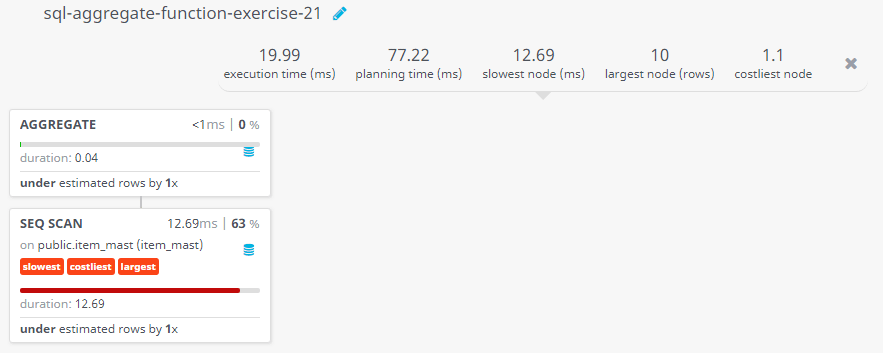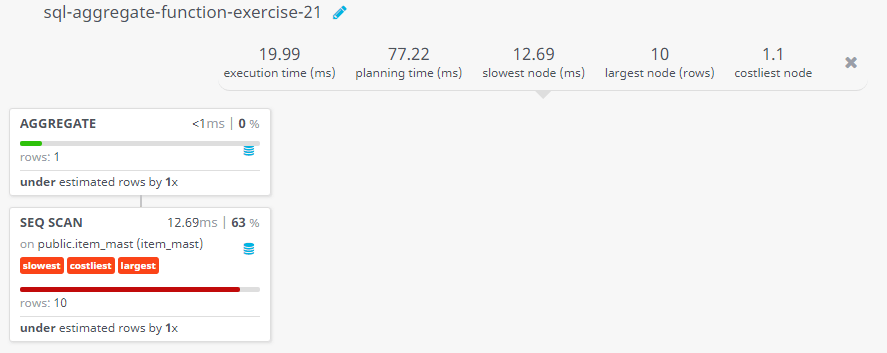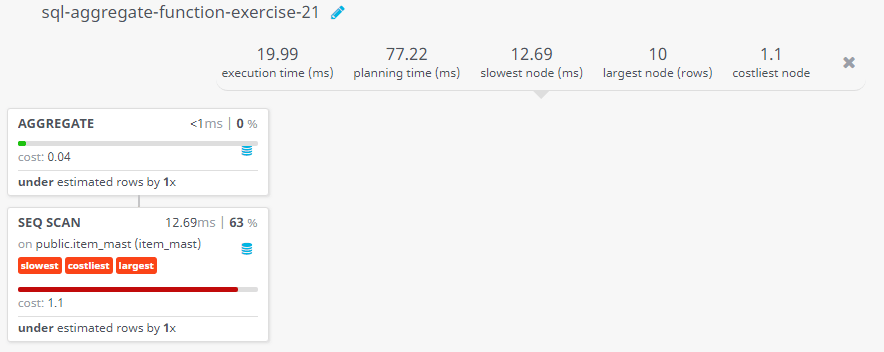﻿ SQL Aggregate Function: Calculate the average price of all the products - w3resource# SQL Exercises: Calculate the average price of all the products

## SQL Aggregate Functions: Exercise-21 with Solution

Write a SQL query to calculate the average price of all the products.

Sample table: item_mast

Sample Solution:

``````SELECT AVG(pro_price) AS "Average Price"
FROM item_mast;
``````

Output of the Query:

```Average Price
1435.0000000000000000
```

## Query Visualization:

Duration:Rows:Cost:Have another way to solve this solution? Contribute your code (and comments) through Disqus.

What is the difficulty level of this exercise?

﻿